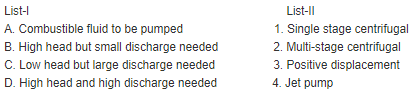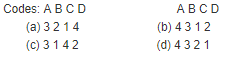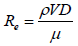Courses

# Dimensional & Model Analysis, Centrifugal Pump - MCQ Test 1

## 20 Questions MCQ Test GATE Mechanical (ME) 2022 Mock Test Series | Dimensional & Model Analysis, Centrifugal Pump - MCQ Test 1

Description
This mock test of Dimensional & Model Analysis, Centrifugal Pump - MCQ Test 1 for Mechanical Engineering helps you for every Mechanical Engineering entrance exam. This contains 20 Multiple Choice Questions for Mechanical Engineering Dimensional & Model Analysis, Centrifugal Pump - MCQ Test 1 (mcq) to study with solutions a complete question bank. The solved questions answers in this Dimensional & Model Analysis, Centrifugal Pump - MCQ Test 1 quiz give you a good mix of easy questions and tough questions. Mechanical Engineering students definitely take this Dimensional & Model Analysis, Centrifugal Pump - MCQ Test 1 exercise for a better result in the exam. You can find other Dimensional & Model Analysis, Centrifugal Pump - MCQ Test 1 extra questions, long questions & short questions for Mechanical Engineering on EduRev as well by searching above.
QUESTION: 1

Solution:

Ans. (a)

QUESTION: 2

Solution:

Ans. (b)

QUESTION: 3

### Assertion (A): Pump lifts water from a lower level to a higher level.Reason (R): In pump, mechanical energy is converted into pressureenergy.

Solution:

Ans. (a)

QUESTION: 4

Match List-I (Industrial needs) with List-II (Type of pump) and select the correct answer using the codes given below the Lists:Solution:

Ans. (b)

QUESTION: 5

The correct sequence of the centrifugal pump components through which the fluid flows is:

Solution:

Ans. (b)

QUESTION: 6

What will be the shape of the velocity triangle at the exit of a radial bladed centrifugal impeller, taking into account slip?

Solution:

Ans. (d)

QUESTION: 7

The power absorbed by a hydraulic pump is directly proportional towhich one of the following?
(Where N is the rotational speed of the pump)

Solution:

Ans. (c)

QUESTION: 8

Consider the following statements pertaining to a centrifugal pump:
1. The manometric head is the head developed by the pump.
2. The suction pipe has, generally, a larger diameter as compared tothe discharge pipe.
3. The suction pipe is provided with a foot valve and a strainer.
4. The delivery pipe is provided with a foot valve and a strainer.
Of these statements:

Solution:

Ans. (c)

QUESTION: 9

A pump running at 1000 RPM consumes 1 kW and generates head of 10m of water. When it is operated at 2000 RPM, its power consumptionand head generated would be:

Solution:

Ans. (d)

QUESTION: 10

A centrifugal pump gives maximum efficiency when its blades are:

Solution:

Ans. (b)

QUESTION: 11

The vanes of a centrifugal pump are generally

Solution:

Ans. (a)

QUESTION: 12

Assertion (A): Multi-stage centrifugal pumps are only of the radial flowtype.
Reason (R): In a multi-stage centrifugal pump, two or more impellersare keyed to a single shaft and enclosed in the same casing, the radialinlet to successive impellers being made through guide vanes.

Solution:

Ans. (b)

QUESTION: 13

If the number of fundamental dimensions equals 'm', then the repeating variables shall be equal to:

Solution:

Ans. (c)

QUESTION: 14

The square root of the ratio of inertia force to gravity force is called

Solution:

Ans. (b)

QUESTION: 15

In flow through a pipe, the transition from laminar to turbulent flowdoes not depend on

Solution:

Ans. (d)QUESTION: 16

Given power 'P' of a pump, the head 'H' and the discharge 'Q' and the specific weight 'w' of the liquid, dimensional analysis would lead to the result that 'P' is proportional to:

Solution:

Ans. (d)

QUESTION: 17

If the number of fundamental dimensions equals 'm', then the repeating variables shall be equal to:

Solution:

Ans. (c)

QUESTION: 18

Consider the following statements:
1. Dimensional analysis is used to determine the number of variables involved in a certain phenomenon
2. The group of repeating variables in dimensional analysis should include all the fundamental units.
3. Buckingham's π theorem stipulates the number of dimensionless groups for a given phenomenon.
4. The coefficient in Chezy's equation has no dimension.
Which of these are correct?

Solution:

Ans. (d) 1 and 4 are wrong, coefficient in Chezy's equation has dimension [L1/2T-1]

QUESTION: 19

Which one of the dimensionless numbers identifies the compressibility effect of a fluid?

Solution:

Ans. (c)

QUESTION: 20

It is observed in a flow problem that total pressure, inertia and gravityforces are important. Then, similarly requires that

Solution:

Ans. (c)